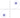## 曲率均匀变化的曲线

Sun, 29th December 2019Edit on Github曲率curvature

# 问题提出

2013年8月，hujunhua提问 :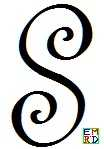# 详细内容

wayne很快给出了一个极坐标微分方程 :

$\rho^{\prime}(\theta )^2+\rho(\theta )^2=\frac{1}{A \theta +B}$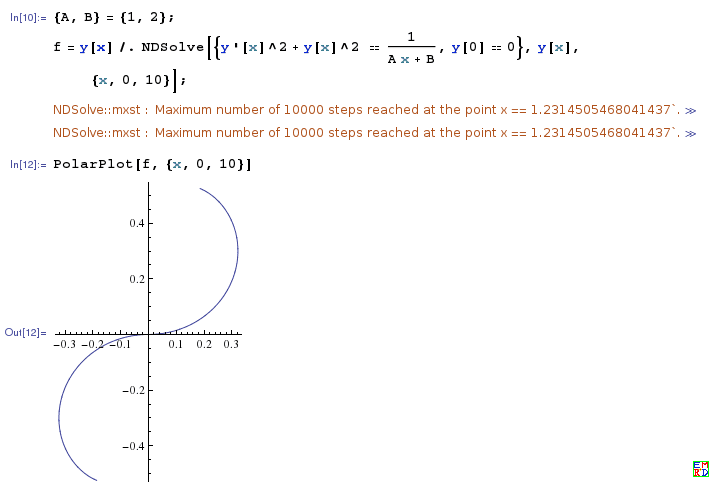$dk=\frac{ds}{\lambda}$$\lambda$为常数） 积分得 $k=\frac{s}{\lambda}$， 将曲率的定义式$k=\frac{d\theta}{ds}$代入得 $\lambda d\theta=s ds$, 积分得到$\lambda\times \theta=\frac12s^2$, 当$s\gt 0$时即为 $s=\sqrt{2\lambda\theta}$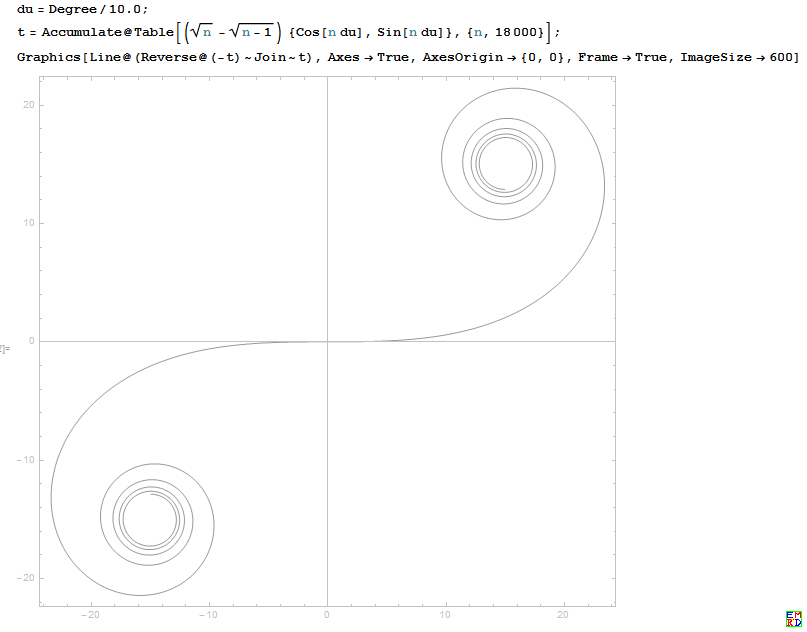zgg__ 搜索到了Cornu Spiral

wayne进一步求解
$2\lambda\theta=s^2 =(\lambda\frac{d\theta}{ds})^2$
$\theta=s^2 =(\frac{\lambda}2)(\frac{d\theta}{ds})^2$

$dy =\frac{p dp}{(\tan^{-1}(p))^{\frac12}(1+p^2)^{\frac32}}$
$dx =\frac{dy}p=\frac{dp}{(\tan^{-1}(p))^{\frac12}(1+p^2)^{\frac32}}$

$x=\sqrt{2 \pi } \text{FresnelC}(\sqrt{\frac{2}{\pi }} \sqrt{\tan ^{-1}(p)})$
$y=\sqrt{2 \pi } \text{FresnelS}(\sqrt{\frac{2}{\pi }} \sqrt{\tan ^{-1}(p)})$

$x=\sqrt{2 \pi } \text{FresnelC}(\sqrt{\frac{2\theta}{\pi }})$
$y=\sqrt{2 \pi } \text{FresnelS}(\sqrt{\frac{2\theta}{\pi }})$
$\theta$即是前面提及的切线的倾角。 跟hujunhua前面提到的羊角螺线完全吻合！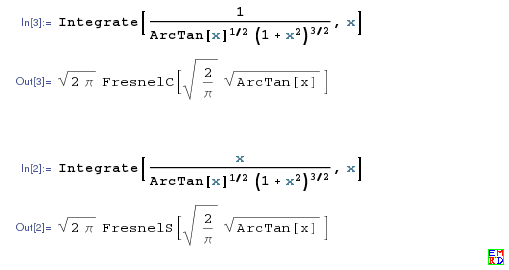hujunhua进一步做变量替换 :

$\begin{cases} d x=\cos\theta\cdot d s\\ d y=\sin\theta\cdot d s \end{cases}$

$\begin{cases} d x=\cos\frac{s^2}{2\lambda}\cdot d s\\\\ d y=\sin\frac{s^2}{2\lambda}\cdot d s \end{cases}$

Integrate[cos(s^2/(2 \[Lambda])),{s,0,\[Infinity]},Assumptions->\[Lambda]>0]

$\{\frac{\sqrt{\lambda\pi}}2,\frac{\sqrt{\lambda\pi}}2\}$$\{-\frac{\sqrt{\lambda\pi}}2,-\frac{\sqrt{\lambda\pi}}2\}$

282842712474表示用复数计算会更加方便

Github